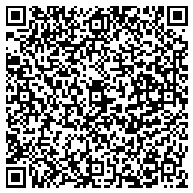### About: List of things named after Pierre de Fermat

an Entity references as follows:

This is a list of things named after Pierre de Fermat, a French amateur mathematician.(This list is incomplete; you can help by expanding it.) * Fermat–Apollonius circle * Fermat–Catalan conjecture * Fermat cubic * Fermat curve * Fermat number * Fermat point * Fermat polygonal number theorem * * Fermat primality test * Fermat Prize * Fermat pseudoprime * Fermat quintic threefold * Fermat quotient * Fermat's difference quotient * Fermat's factorization method * Fermat's last theorem * Fermat's little theorem * Fermat's principle * Fermat's spiral * Fermat's theorem (stationary points) * Fermat's theorem on sums of two squaresGraph IRICount
http://dbpedia.org41 triples
Faceted Search & Find service v1.17_git51

Alternative Linked Data Documents: PivotViewer | iSPARQL | ODE     Raw Data in: CXML | CSV | RDF ( N-Triples N3/Turtle JSON XML ) | OData ( Atom JSON ) | Microdata ( JSON HTML) | JSON-LD    About